﻿ 基于实测数据的阿克苏光伏电站相关性分析

# 基于实测数据的阿克苏光伏电站相关性分析Relevance Analysis of Aksu Photovoltaic Power Station Based on Measured Data

Abstract: Uncertainty, intermittence and fluctuation of photovoltaic power generation will cause photovoltaic power generation to abandon light, and mastering its characteristics is an important basis for improving absorption. In this paper, the correlation of photovoltaic power plants is analyzed based on measured data. Firstly, the calculation method of the correlation between the output of the same photovoltaic power station at different time periods and the output of the same photovoltaic power station at different time periods is given. Secondly, the influence of cloud factor and geographic location on the output correlation of photovoltaic power station is analyzed theoretically. Finally, taking Aksu photovoltaic power station in Xinjiang as an example, the influence of cloud factor and geographic location on the output correlation of the same photovoltaic power station and the output correlation of different photovoltaic power stations are illustrated by the measured data.

1. 引言

2. 光伏电站出力相关性的计算方法

2.1. 同一光伏电站不同时段出力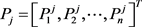(1)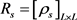(2)

${\rho }_{s}=r\left({P}^{\alpha },{P}^{\beta }\right)=\frac{\underset{i=1}{\overset{n}{\sum }}\left({P}_{i}^{\alpha }-\stackrel{¯}{{P}^{\alpha }}\right)\left({P}_{i}^{\beta }-\stackrel{¯}{{P}^{\beta }}\right)}{\sqrt{\underset{i=1}{\overset{n}{\sum }}{\left({P}_{i}^{\alpha }-\stackrel{¯}{{P}^{\alpha }}\right)}^{2}\underset{i=1}{\overset{n}{\sum }}{\left({P}_{i}^{\beta }-\stackrel{¯}{{P}^{\beta }}\right)}^{2}}}$ (3)

2.2. 同一时段不同光伏电站出力

${R}_{T}={\left[{\rho }_{T}\right]}_{M×M}$ (4)

${\rho }_{T}=r\left({P}_{T}^{f},{P}^{g}\right)=\frac{\underset{i=1}{\overset{n}{\sum }}\left({P}_{i+T}^{f}-\stackrel{¯}{{p}^{f}}\right)\left({P}_{i}^{g}-\stackrel{¯}{{P}^{g}}\right)}{\sqrt{\underset{i=1}{\overset{n}{\sum }}{\left({P}_{i+T}^{f}-\stackrel{¯}{{p}^{f}}\right)}^{2}\underset{i=1}{\overset{n}{\sum }}{\left({P}_{i}^{g}-\stackrel{¯}{{P}^{g}}\right)}^{2}}}$ (5)

3. 光伏电站出力相关性的影响因素理论分析

3.1. 云量因子

$x=\left[0,\cdots ,0,{\gamma }_{1}{x}_{1},{\gamma }_{2}{x}_{2},{\gamma }_{3}{x}_{3},\cdots ,{\gamma }_{n-2}{x}_{n-2},{\gamma }_{n-1}{x}_{n-1},{\gamma }_{n}{x}_{n},0,\cdots ,0\right]$ (6)(7)

${y}^{\prime }=\left[0,\cdots ,0,{\beta }_{1}{{y}^{\prime }}_{1},{\beta }_{2}{{y}^{\prime }}_{2},{\beta }_{3}{{y}^{\prime }}_{3},\cdots ,{\beta }_{n-2}{{y}^{\prime }}_{n-2},{\beta }_{n-1}{{y}^{\prime }}_{n-1},{\beta }_{n}{{y}^{\prime }}_{n},0,\cdots ,0\right]$ (8)

$\rho \left(x,y\right)=\frac{\left(N-n\right)\stackrel{¯}{x}\cdot \stackrel{¯}{y}+\underset{i=1}{\overset{n}{\sum }}\left({\gamma }_{i}{x}_{i}-\stackrel{¯}{x}\right)\left({\alpha }_{i}{y}_{i}-\stackrel{¯}{y}\right)}{\sqrt{\left(\left(N-n\right){\left(0-\stackrel{¯}{x}\right)}^{2}+\underset{i=1}{\overset{n}{\sum }}{\left({\gamma }_{i}{x}_{i}-\stackrel{¯}{x}\right)}^{2}\right)\cdot \left(\left(N-n\right){\left(0-\stackrel{¯}{y}\right)}^{2}+\underset{i=1}{\overset{n}{\sum }}{\left({\alpha }_{i}{y}_{i}-\stackrel{¯}{y}\right)}^{2}\right)}}$ (9)

$\rho \left(x,{y}^{\prime }\right)=\frac{\left(N-n\right)\stackrel{¯}{x}\cdot {\stackrel{¯}{y}}^{\prime }+\underset{i=1}{\overset{n}{\sum }}\left({\gamma }_{i}{x}_{i}-\stackrel{¯}{x}\right)\left({\beta }_{i}{{y}^{\prime }}_{i}-{\stackrel{¯}{y}}^{\prime }\right)}{\sqrt{\left(\left(N-n\right){\left(0-\stackrel{¯}{x}\right)}^{2}+\underset{i=1}{\overset{n}{\sum }}{\left({\gamma }_{i}{x}_{i}-\stackrel{¯}{x}\right)}^{2}\right)\cdot \left(\left(N-n\right){\left(0-{\stackrel{¯}{y}}^{\prime }\right)}^{2}+\underset{i=1}{\overset{n}{\sum }}{\left({\beta }_{i}{{y}^{\prime }}_{i}-{\stackrel{¯}{y}}^{\prime }\right)}^{2}\right)}}$ (10)

3.2. 地理位置

$x=\left[0,\cdots ,0,{x}_{1},{x}_{2},{x}_{3},\cdots ,{x}_{n-2},{x}_{n-1},{x}_{n},0,0,\cdots ,0\right]$ (11)

$y=\left[0,\cdots ,0,0,{y}_{1},{y}_{2},\cdots ,{y}_{n-1},{y}_{n},0,0,\cdots ,0\right]$ (12)

${y}^{\prime }=\left[0,\cdots ,0,0,0,{{y}^{\prime }}_{1},\cdots ,{{y}^{\prime }}_{n},0,0,\cdots ,0\right]$ (13)

$\rho \left(x,y\right)=\frac{\begin{array}{l}\text{}\left(N-n-2\right)\stackrel{¯}{x}\cdot \stackrel{¯}{y}+\left({x}_{1}-\stackrel{¯}{x}\right)\left(0-\stackrel{¯}{y}\right)+\left({x}_{2}-\stackrel{¯}{x}\right)\left({y}_{1}-\stackrel{¯}{y}\right)\\ +\underset{i=3}{\overset{n}{\sum }}\left({x}_{i}-\stackrel{¯}{x}\right)\left({y}_{i-1}-\stackrel{¯}{y}\right)\text{}+\left(0-\stackrel{¯}{x}\right)\left({y}_{n}-\stackrel{¯}{y}\right)+\left(0-\stackrel{¯}{x}\right)\left(0-\stackrel{¯}{y}\right)\end{array}}{\sqrt{\left(\left(N-n\right){\left(0-\stackrel{¯}{x}\right)}^{2}+\underset{i=1}{\overset{n}{\sum }}{\left({x}_{i}-\stackrel{¯}{x}\right)}^{2}\right)\cdot \left(\left(N-n\right){\left(0-\stackrel{¯}{y}\right)}^{2}+\underset{i=1}{\overset{n}{\sum }}{\left({y}_{i}-\stackrel{¯}{y}\right)}^{2}\right)}}$ (14)

$\rho \left(x,{y}^{\prime }\right)=\frac{\begin{array}{l}\text{}\left(N-n-2\right)\stackrel{¯}{x}\cdot {\stackrel{¯}{y}}^{\prime }+\left({x}_{1}-\stackrel{¯}{x}\right)\left(0-{\stackrel{¯}{y}}^{\prime }\right)+\left({x}_{2}-\stackrel{¯}{x}\right)\left(0-{\stackrel{¯}{y}}^{\prime }\right)\\ +\underset{i=3}{\overset{n}{\sum }}\left({x}_{i}-\stackrel{¯}{x}\right)\left({{y}^{\prime }}_{i-2}-{\stackrel{¯}{y}}^{\prime }\right)\text{}+\left(0-\stackrel{¯}{x}\right)\left({y}_{n-1}-{\stackrel{¯}{y}}^{\prime }\right)+\left(0-\stackrel{¯}{x}\right)\left({y}_{n}-{\stackrel{¯}{y}}^{\prime }\right)\end{array}}{\sqrt{\left(\left(N-n\right){\left(0-\stackrel{¯}{x}\right)}^{2}+\underset{i=1}{\overset{n}{\sum }}{\left({x}_{i}-\stackrel{¯}{x}\right)}^{2}\right)\cdot \left(\left(N-n\right){\left(0-{\stackrel{¯}{y}}^{\prime }\right)}^{2}+\underset{i=1}{\overset{n}{\sum }}{\left({y}_{i}-{\stackrel{¯}{y}}^{\prime }\right)}^{2}\right)}}$ (15)

$\Delta \rho =\rho \left(x,y\right)-\rho \left(x,{y}^{\prime }\right)=\frac{{x}_{2}{y}_{1}+\left({y}_{n-1}-{y}_{1}\right)\stackrel{¯}{x}}{\sqrt{\left(\left(N-n\right){\left(0-\stackrel{¯}{x}\right)}^{2}+\underset{i=1}{\overset{n}{\sum }}{\left({x}_{i}-\stackrel{¯}{x}\right)}^{2}\right)\cdot \left(\left(N-n\right){\left(0-\stackrel{¯}{y}\right)}^{2}+\underset{i=1}{\overset{n}{\sum }}{\left({y}_{i}-\stackrel{¯}{y}\right)}^{2}\right)}}$ (16)

4. 实测数据光伏电站出力相关性分析Table 1. Basic information of photovoltaic power station

4.1. 云量因子对相关性的影响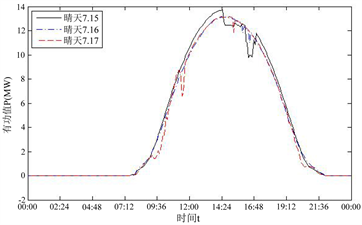Figure 1. Output curve on sunny day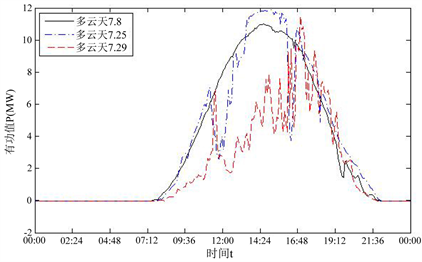Figure 2. Output curve in cloudy day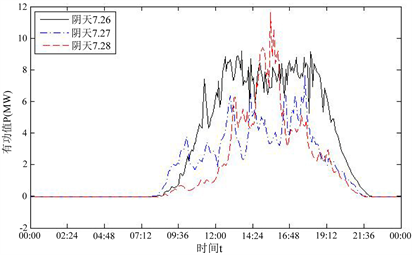Figure 3. Output curve in overcast dayTable 3. Coefficient of correlation under different cloud coverTable 4. Correlation coefficient statistics

4.2. 地理位置对相关性的影响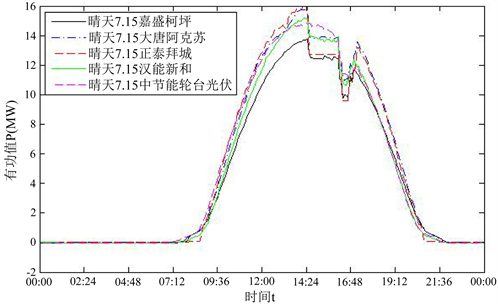Figure 4. Output curves of five power plants on sunny day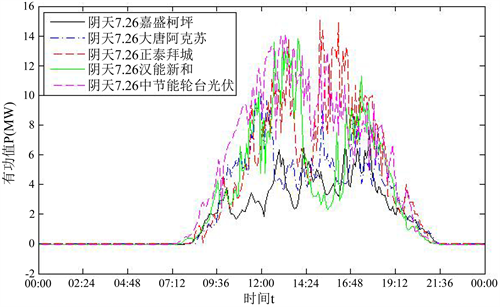Figure 5. Output curves of five power plants on cloudy day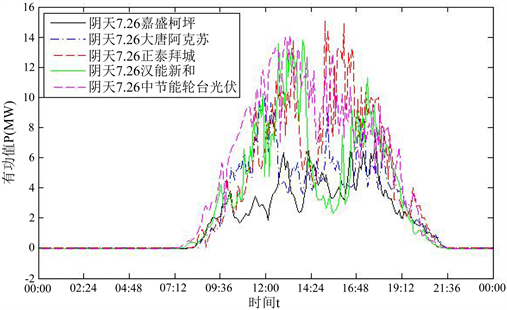Figure 6. Output curves of five power plants on overcast dayTable 5. Coefficient of correlation and delay of geographical location considering delay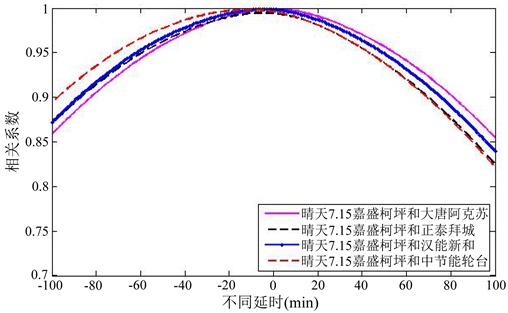Figure 7. Delay characteristics of sunny day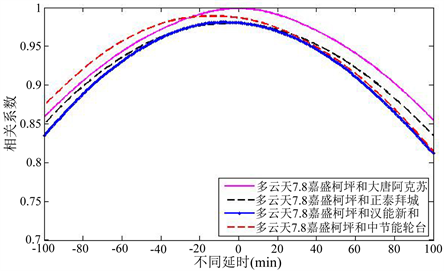Figure 8. Delay characteristics of cloudy day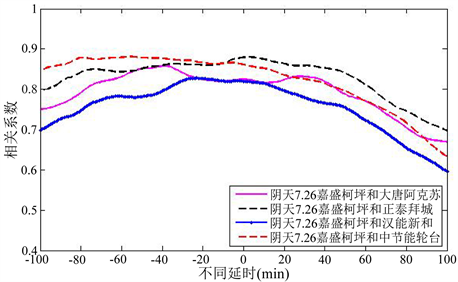Figure 9. Delay characteristics of overcast day

5. 结论

1) 晴天与晴天的相关系数的最小值大于除了晴天与多云天组合之外的任意组合的最大值，表明同一光伏电站出力相关性最强，原因在于两者的云量因子接近且干扰因素较小。

2) 晴天和多云天情况下，参考电站的出力序列的起始点和终止点晚于比较电站，比较电站的出力序列只有经过延时，不同地理位置的光伏电站出力相关性才能达到最强。

 Woyte, A., Van, V., Belmans, R., et al. (2006) Voltage Fluctuations on Distribution Level Introduced by Photovoltaic Systems. IEEE Transactions on Energy Conversion, 21, 202-209.
https://doi.org/10.1109/TEC.2005.845454

 张程飞, 刘纯, 王跃峰, 等. 基于模糊多目标优化的电网日前风电接纳能力评估模型[J]. 电网技术, 2015, 39(2): 426-431.

 田建芳, 毛亚珊, 翟桥柱, 等. 基于风电消纳能力评估的安全约束经济调度方法[J]. 电网技术, 2015, 39(9): 2398-2403.

 Perez, R., Kivalov, S., Schlemmer, J., et al. (2012) Short-Term Irradiance Variability: Preliminary Estimation of Station Pair Correlation as a Function of Distance. Solar Energy, 86, 2170-2176.
https://doi.org/10.1016/j.solener.2012.02.027

 Hollands, K.G.T. and Huget, R.G. (1990) A Probability Densitity Function for the Clearness Index, with Applications. Solar Energy, 30, 195-209.

 Graham, V.A. and Hollands, K.G.T. (1990) A Method to Generate Synthetic Hourly Solar Radion Globally. Solar Energy, 44, 333-341.

 姚荃. 考虑不确定因素的光伏发电出力预测[D]: [硕士学位论文]. 北京: 华北电力大学, 2012.

 张曦, 康重庆, 张宁, 黄越辉, 刘纯, 徐健飞. 太阳能光伏发电的中长期随机特性分析[J]. 电力系统自动化, 2014, 38(6): 6-13.

 张慧玲, 喻洁, 韩红卫, 冯其芝. 间歇性新能源发电相关性分析[J]. 电气自动化, 2015, 37(5): 30-34.

 汪海瑛, 白晓民. 并网光伏的短期运行备用评估[J]. 电力系统自动化, 2013, 37(5): 55-60.

 李芝娟. 基于实测数据的风电场群功率特性研究[D]: [硕士学位论文]. 北京: 华北电力大学, 2015.

 崔杨, 李焕奇, 张节潭, 严干贵, 穆钢, 王茂春. 计及功率相关性的光伏电站群置信容量评估方法[J]. 太阳能学报, 2017, 38(4): 1039-1044.

Top